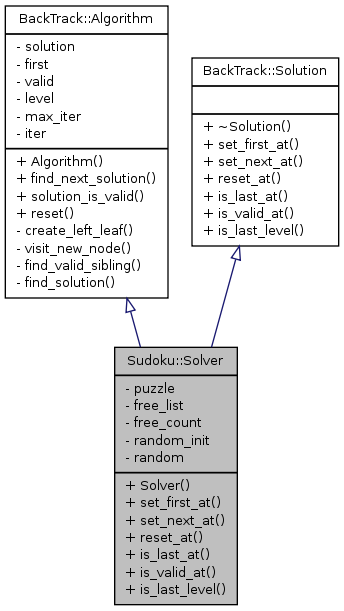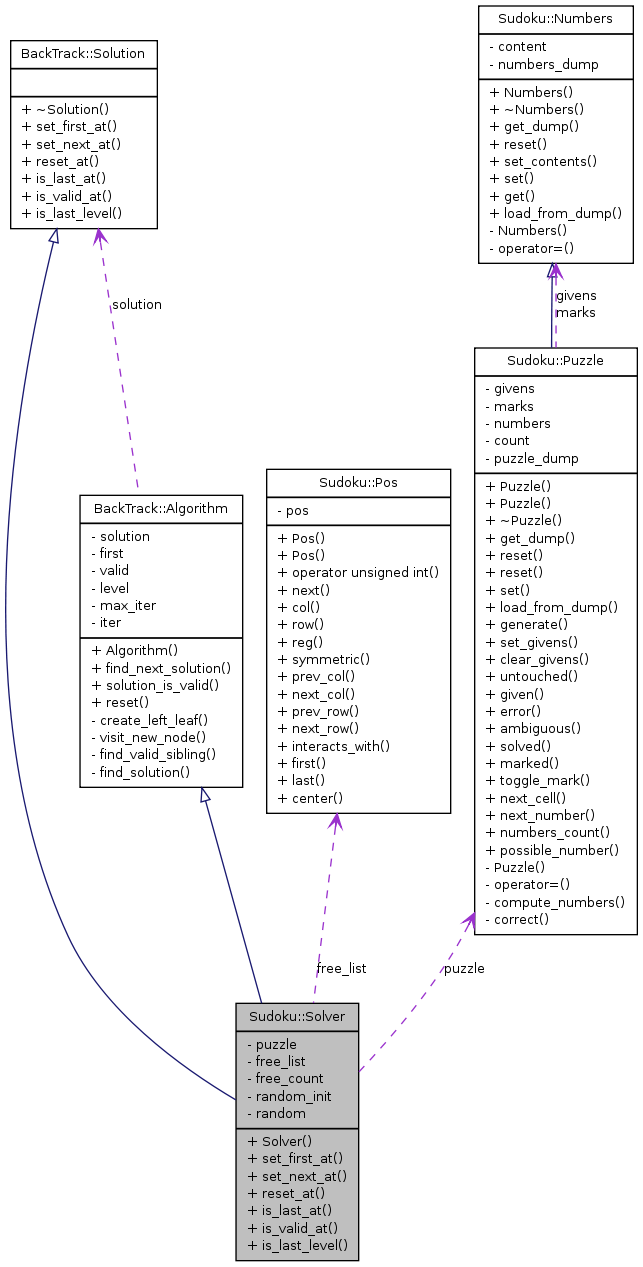# Sudoku::Solver Class Reference

Implementation of a backtracking algorithm to solve Sudoku puzzles. More...

`#include <solver.h>`

Inheritance diagram for Sudoku::Solver:[legend]
Collaboration diagram for Sudoku::Solver:[legend]

List of all members.

## Public Member Functions

Solver (Puzzle &puzzle, bool random_init=false, unsigned int max_iter=0)
Constructor.
virtual void set_first_at (unsigned int level)
Set the element to the first sibling.
virtual void set_next_at (unsigned int level)
Set the element to the next sibling.
virtual void reset_at (unsigned int level)
Reset the element.
virtual bool is_last_at (unsigned int level) const
Check if the element is set to the last sibling.
virtual bool is_valid_at (int level) const
Check if the element is valid (following elements ignored).
virtual bool is_last_level (int level) const
Check if the level is the last possible level.

## Private Attributes

Puzzlepuzzle
Pos free_list [SDIM]
unsigned int free_count
bool random_init
bool random

## Detailed Description

Implementation of a backtracking algorithm to solve Sudoku puzzles.

The algorithm tries to set numbers in all free cells of the puzzle sticking to the rules of Sudoku puzzles.

There are three ways to use this class:

1. Solve a Sudoku puzzle with some numbers given:

```    Puzzle puzzle(36); // Generate a random puzzle with 36 givens.
Solver solver(puzzle);
solver.find_next_solution();
if (solver.solution_is_valid())
{
// Do something with the puzzle.
...
}
solver.find_next_solution();
bool more_than_one_solution = solver.solution_is_valid();
```

2. Search a random Sudoku solution:

```    Puzzle puzzle; // Generate an empty puzzle without givens.
Solver solver(puzzle, true);
solver.find_next_solution();
if (solver.solution_is_valid())
{
// Do something with the puzzle.
...
}
```

3. Search all Sudoku solutions:

```    Puzzle puzzle; // Generate an empty puzzle without givens.
Solver solver(puzzle);
solver.find_next_solution();
while (solver.solution_is_valid())
{
// Do something with the puzzle.
...
solver.find_next_solution();
}
```

Definition at line 83 of file solver.h.

## Constructor & Destructor Documentation

 Solver::Solver ( Puzzle & puzzle, bool random_init = `false`, unsigned int max_iter = `0` )

Constructor.

Definition at line 34 of file solver.cpp.

References Sudoku::Pos::first(), free_count, Sudoku::Puzzle::given(), and Sudoku::Pos::last().

## Member Function Documentation

 bool Solver::is_last_at ( unsigned int level ) const` [virtual]`

Check if the element is set to the last sibling.

Implements BackTrack::Solution.

Definition at line 88 of file solver.cpp.

References free_count, free_list, Sudoku::Puzzle::next_number(), and puzzle.

 bool Solver::is_last_level ( int level ) const` [virtual]`

Check if the level is the last possible level.

Implements BackTrack::Solution.

Definition at line 104 of file solver.cpp.

References free_count.

 bool Solver::is_valid_at ( int level ) const` [virtual]`

Check if the element is valid (following elements ignored).

Implements BackTrack::Solution.

Definition at line 95 of file solver.cpp.

References Sudoku::Puzzle::error(), free_count, free_list, puzzle, and Sudoku::Puzzle::solved().

 void Solver::reset_at ( unsigned int level ) ` [virtual]`

Reset the element.

Implements BackTrack::Solution.

Definition at line 80 of file solver.cpp.

References free_count, free_list, puzzle, random, and Sudoku::Puzzle::set().

 void Solver::set_first_at ( unsigned int level ) ` [virtual]`

Set the element to the first sibling.

Implements BackTrack::Solution.

Definition at line 44 of file solver.cpp.

 void Solver::set_next_at ( unsigned int level ) ` [virtual]`

Set the element to the next sibling.

Implements BackTrack::Solution.

Definition at line 70 of file solver.cpp.

References free_count, free_list, Sudoku::Puzzle::next_number(), puzzle, and Sudoku::Puzzle::set().

## Member Data Documentation

 unsigned int Sudoku::Solver::free_count` [private]`
 Pos Sudoku::Solver::free_list[SDIM]` [private]`

Definition at line 86 of file solver.h.

Referenced by is_last_at(), is_valid_at(), reset_at(), set_first_at(), and set_next_at().

 Puzzle& Sudoku::Solver::puzzle` [private]`

Definition at line 85 of file solver.h.

Referenced by is_last_at(), is_valid_at(), reset_at(), set_first_at(), and set_next_at().

 bool Sudoku::Solver::random` [private]`

Definition at line 88 of file solver.h.

Referenced by reset_at(), and set_first_at().

 bool Sudoku::Solver::random_init` [private]`

Definition at line 88 of file solver.h.

Referenced by set_first_at().

The documentation for this class was generated from the following files:
Generated on Mon Apr 5 17:01:13 2010 for VDR plugin 'Sudoku' by1.6.3